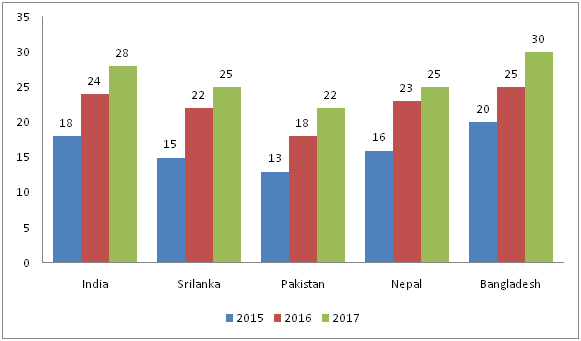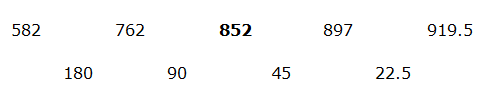# SBI PO Prelims Quantitative Aptitude Questions 2019 – Day 20

SBI PO 2019 Notification will be expected soon. It is one of the most expected recruitment among the banking aspirants. Every year the exam pattern for SBI PO has been changing. Depends upon the changing of exam pattern the questions are quite harder compare to the previous year. So the questions are in high level than the candidate’s assumption.

As per the latest trend, our IBPS Guide is providing the updated New Exam Pattern Quantitative Aptitude questions for SBI PO 2019 Day 20. Our Skilled experts were mounting the questions based on the aspirant’s needs. So candidates shall start your preparation and practice on daily basis with our SBI PO pattern quantitative aptitude questions 2019 day 20. Start your effective preparation from the right beginning to get success in upcoming SBI PO 2019.

[WpProQuiz 5381]

### Click Here for SBI PO Pre 2019 High-Quality Mocks Exactly on SBI Standard

Direction (1- 5): Find the wrong term in the following number series

1) 356, 354, 362, 386, 446, 566

a) 446

b) 566

c) 354

d) 386

e) 362

2) 22, 24, 32, 70, 168, 511

a) 168

b) 24

c) 70

d) 511

e) 32

3) 730, 366, 184, 92, 47.5, 24.75

a) 47.5

b) 366

c) 730

d) 92

e) 184

4) 582, 762, 853, 897, 919.5

a) 582

b) 853

c) 762

d) 897

e) 919.5

5) 8, 8, 40, 360, 4675, 79560

a) 360

b) 79560

c) 4675

d) 40

e) 8

Directions (Q. 6 – 10): Study the following information carefully and answer the given questions:

The following bar graph shows the gross national income (In billion dollars) of 5 different countries in 3 different years.6) Find the difference between the gross national income of India to that of Nepal in all the given years together?

a) 8 billion dollars

b) 6 billion dollars

c) 10 billion dollars

d) 14 billion dollars

1. e) None of these

7) Find the ratio between the gross national income of Srilanka in 2015 and 2017 together to that of the gross national income of Bangladesh in 2016 and 2017 together?

a) 5: 9

b) 13: 17

c) 25: 33

d) 8: 11

e) None of these

8) Find the average gross national income in 2017 in all the given countries together?

a) 26 billion dollars

b) 22 billion dollars

c) 20 billion dollars

d) 28 billion dollars

e) None of these

9) The gross national income of Srilanka, Pakistan and Nepal together in 2016 is approximately what percentage of the gross national income of India, Nepal and Bangladesh together in 2015?

a) 100 %

b) 135 %

c) 120 %

d) 90 %

e) 75 %

10) The gross national income of Pakistan in all the given years together is approximately what percentage more/less than the gross national income of Srilanka in all the given years together?

a) 40 % more

b) 15 % less

c) 40 % less

d) 15 % more

e) 25 % less

Direction (1-5) :

The correct series is,

356, 356, 362, 386, 446, 566

The pattern is, +13 – 1, +23 – 2, +33 – 3, +43 – 4, +53 – 5,….

The wrong term is, 354

The correct series is,

22, 24, 32, 70, 167, 511

The pattern is, *1 + 2, *1.5 – 4, *2 + 6, *2.5 – 8, *3 + 10,….

The wrong term is, 168

The correct series is,

730, 366, 184, 93, 47.5, 24.75

The pattern is, ÷ 2 + 1

The wrong term is, 92The correct series is,

582, 762, 852, 897, 919.5

The pattern is, the difference of two numbers ÷ 2

The wrong term is, 853

The correct series is,

8, 8, 40, 360, 4680, 79560

The pattern is, *1, *5, *9, *13, *17,…

The wrong term is, 4675

Direction (6-10 ):

The gross national income of India in all the given years together

= > 18 + 24 + 28 = 70 billion dollars

The gross national income of Nepal in all the given years together

= > 16 + 23 + 25 = 64 billion dollars

Required difference = 70 – 64 = 6 billion dollars

The gross national income of Srilanka in 2015 and 2017 together

= > 15 + 25 = 40 billion dollars

The gross national income of Bangladesh in 2016 and 2017 together

= > 25 + 30 = 55 billion dollars

Required ratio = 40: 55 = 8: 11

The average gross national income in 2017 in all the given countries together

= > (28 + 25 + 22 + 25 + 30)/5

= > 130/5 = 26 billion dollars

The gross national income of Srilanka, Pakistan and Nepal together in 2016

= > 22 + 23 + 18 = 63 billion dollars

The gross national income of India, Nepal and Bangladesh together in 2015

= > 18 + 16 + 20 = 54 billion dollars

Required % = (63/54)*100 = 116.67 % = 120 %

The gross national income of Pakistan in all the given years together

= > 13 + 18 + 22 = 53 billion dollars

The gross national income of Srilanka in all the given years together

= > 15 + 22 + 25 = 62 billion dollars

Required % = [(62 – 53)/62]*100 = 14.5 % = 15 % less

5 1 vote
Rating